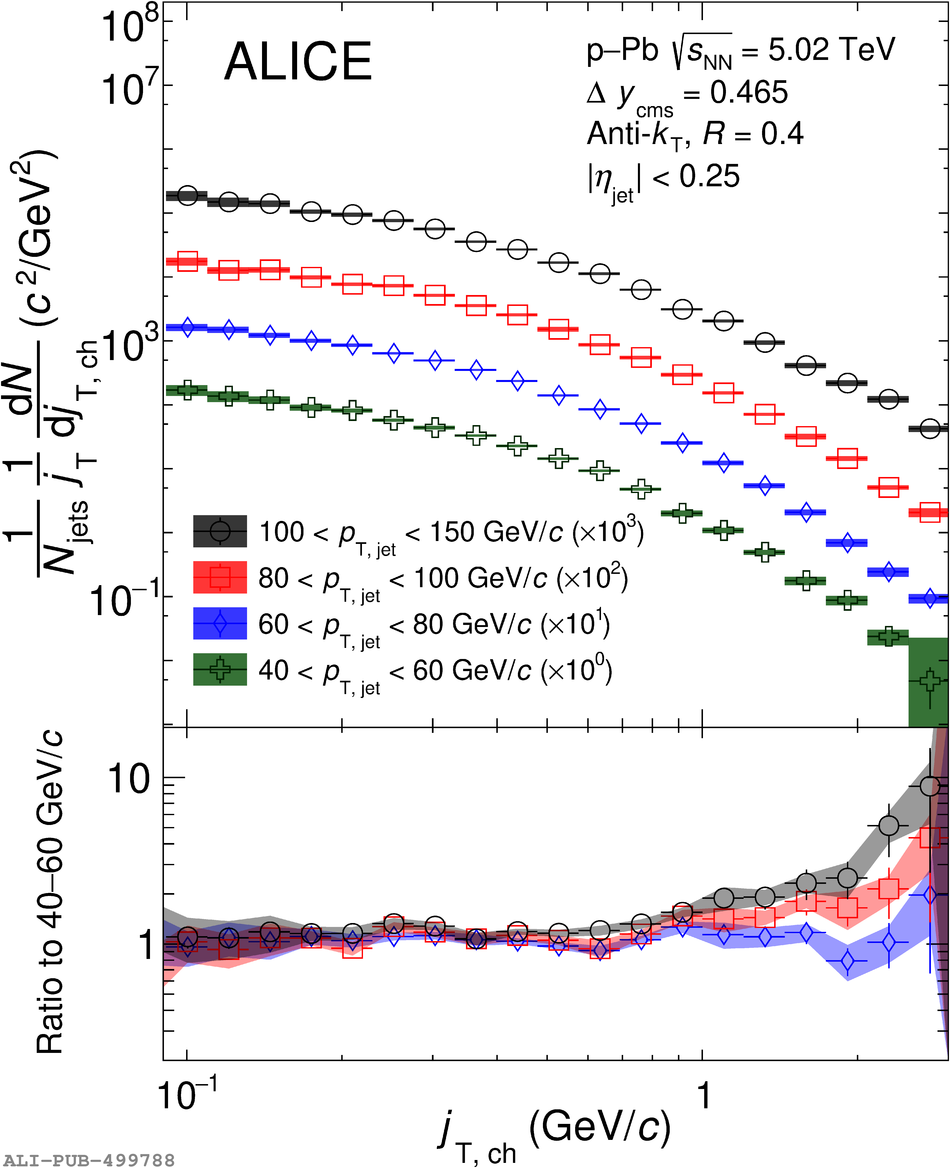# Figure 2

 The $\jt$ distributions of charged particles in $R = 0.4$ anti-$k_\mathrm{T}$ jets as measured in $\pPb$ collisions at $\sqrtSnnE{5.02}$ for different ranges of $\ptjet$. The centre-of-mass rapidity is shifted by $\Delta y = 0.465$ in the direction of the proton beam. The bottom panel shows ratios of the $\jt$ distributions with respect to that in \unit[\$40